Get instant CPM homework help from the best tutors at TutorEye

Our homework helpers have meticulous writing and research skills. Hire one now and invest your saved time in focusing on understanding the concepts.

## College Preparatory Mathematics

CPM stands for College Preparatory Mathematics. It is the most effective course that helps the students to prepare for their SATs and other competitive examinations. There are three different kinds of CPM modules that are discussed below, or we can say that you might face issues in these courses and might require CPM homework help on these.

## What are the different types of CPM?

There are three different kinds of CPM. Let’s spend some time to understand what one learns under each of these core connections five-year college mathematics courses.

### Core Connection 1 (CC1):

CC1 is the 1st course in the full series of CPM courses. The focus of the course is to strengthen the foundation of mathematical concepts on topics such as time, speed, distance, volume, area, percent, fractions, and decimals; simplify variable expressions and more. This course prepares the middle school students for the advanced math of high school. Therefore, it is critical to get the correct guidance for your child as an expert help can take away the extra pressure & enable the child to work with the top part of their brain when dealing with CC1 homework questions. Our experts are available 24*7 to provide CPM homework help to students taking CC1.

### Core Connection 2 (CC2):

CC2 is the second course in the full series of CPM courses. It focuses on developing and reinforcing the geometry learn by students in CC1 course. Students taking this course learn how to identify the relationships between concepts. For example, students learn how to apply discounts to a particular product, markup, find coefficient of fractions, solve linear equations with two variables, find the perimeter and area of compound shapes, pair angles with each other, decrease or increase interest rates.

As the complexity of math concepts studied by students increase so does the fear of falling behind in class or not able to solve the homework questions independently. This is where CPM homework helpers from TutorEye can helps students analyze, understand, and solve their homework assignments with ease and clarity.

### Core Connection 3 (CC3):

CC3 is the 3rd course in a 5-year sequence of CPM courses. Students in core connections 3 learn how to establish connections between multiple representations of functions and drawing statistical conclusions from the data points available. Common items studied under CC3 include linear functions, tables and graphs, logarithms, inversions, polynomials, slope ratios; scatterplots, data representation and transversality theorem, pythagorean theorem, as well as triangle angle sum theorem.

Being the final one of the list, it is not doubt the most complex of all three and hence students may need additional guidance from time to time with CPM homework help.

CPM or College Preparatory Mathematics is a program that focuses on student objectives and learning outcomes. It introduces them to the concepts of formulas, diagrams, algorithms, and functions

Understanding these concepts is often fascinating for those who have a knack for solving mathematical problems. But for beginners, it can be a tad bit overwhelming and they end up looking for help in assignments.

No doubt, it is considered to be a tough course, the importance of this course cannot be overlooked. Not only does this subject help students in understanding mathematical concepts, but also empowers them with basic knowledge of the requisites that can strengthen their problem-solving capabilities.

One thing that students often struggle with is the correct use of formulas in their CPM homework. This is why they prefer to rely on online sources for help.

Getting in touch with the right helpers can help you improve at your assignments and make you more confident in the respective subject.

## Wondering who can take college prep mathematics?

You will be surprised to know that College Preparatory Mathematics is for everyone. Regardless of the college subjects you pick or later on the career path you select, CPM or  College Preparatory Mathematics can help all students.

As the name suggests, College prep math is closely related to getting students ready for college level math. In Simple words, it means that you are studying the same math but just giving an additional boost to your knowledge and fundamentals of mathematics. By Studying CPM, students don’t need to spend additional time an energy for preparing for college entrance exams like SAT.

## CPM Homework Help - Core Homework Topics

• CPM Homework Help Algebra 1
• CPM Homework Help Algebra 2
• CPM Homework Help Geometry
• CPM Homework Help Statistics
• CPM Homework Help Calculus
• CPM Precalculus Homework Help

## CPM Sample Questions:

Question 1: John has five x^2-tiles, three x- tiles and eight unit tiles, while Malia has three x^2-tiles and six unit tiles. At the end of the class they put their pieces together to give to Ms.Kapoor.

Choose the correct algebraic expression that students give to Ms. Kapoor?

(a) 8x^2+3x+13

(b) 3x^2+8x+14

(c) 8x^2+3x+14

(d) 3x^2+5x+13

Explanation: Algebraic expression will be as follows:
John - 5x^2+3x+8
Malia - 3x^2+6

Question 2: Find the domain and range of the given graph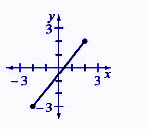(a) Domain: - 2 ≤ x ≤ 2 & Range: - 3 ≤ y ≤ 2

(b) Domain: - 2 < x ≤ 2 & Range: - 3 ≤ y < 2

(c) Domain: - 2 < x < 2 & Range: - 3 < y < 2

(d) Domain: - 2 ≤ x < 2 & Range: - 3 < y ≤ 2

Explanation: Domain is the set of x-coordinate and range is the set of y-coordinates

Question 3: Following the pattern fill all the value of last figure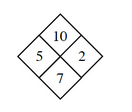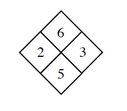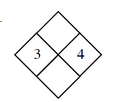(a)(b)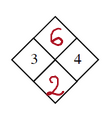(c)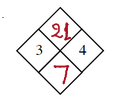(d)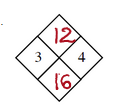Explanation: We noticed that the two side numbers (5&2) when added give the bottom number (5+2=7) and when both the numbers are multiplied it gives the top number (5×2=10)

Question 4: Tom is 8 year older than his brother Jack. The sum of their ages is 64.How old are Jack and Tom?

(a) 28year & 36year

(b) 24year & 32year

(c) 22year & 30year

(d) 20year & 28year

Explanation: Let us assume that age of Jack be x year
then, age of Tom be x+8 year

Question 5: Find the ∠CPM of the given figure?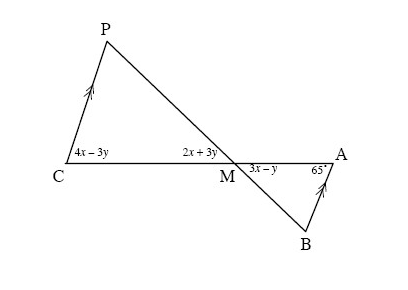(a) 50 degree

(b) 60 degree

(c) 70 degree

(d) 80 degree

Explanation:  ∠PCM = ∠MAB = 65 degree   [∴Alternate angle]

Question 6: Find the measure of angle A in the diagram below:(a) 17.46

(b) 18.46

(c) 18

(d) 17

Explanation: Sin A = Perpendicular/Hypotenuse = 0.3/1

Question 7: What is the probability that x^2 + 7x + k is factorable if 0 ≤ k ≤ 20 and k is an integer?

(a) 19.05

(b) 18.05

(c) 17.05

(d) 20.05

Explanation: First we find all the value of k for which the expression is factorable

Question 8: Eight friends go to the movies.They want to sit together in a row. In how many ways they can be in the row?

(a) 40320

(b) 45360

(c) 35280

(d) 25920

Explanation: There are eight friends. So, they can sit in 8! ways.

Question 9: Solve for x:(a) 11.5

(b) 12.5

(c) 13.5

(d) 10.5

Explanation: Using the definition of logarithm

Question 10: The perimeter of a triangle is 31 cm. Two of its sides have equal length, while the other side is one centimeter shorter than twice the length of the side . How long is each side?

(a) 13,7,7

(b) 14,6,6

(c) 10,11,11

(d) 15,8,8

Explanation: Let the two equal side of triangle be a cm each
And the third side = (2a -1)cm

### How can TutorEye relieve your assignment-related stress?

Our problem solvers are experts in their subjects and understand your requirements well. This helps them deliver the solutions to your questions with great efficiency.

Also, student satisfaction is our prime motive and we strive to provide the best academic help at affordable prices.

Our CPM homework helpers will provide you 100% error-free and authentic solutions for cc1 as well as cc2. Help from professionals will have a great impact on your academics and will improve your overall grades.

## Easy steps to get help in CPM practice problems

At TutorEye, we have Ph.D. and Master’s level subject experts who help you solve your assignment problems. Get detailed solutions to all your questions at a reasonable price.

Follow these simple steps to hire a problem solver-